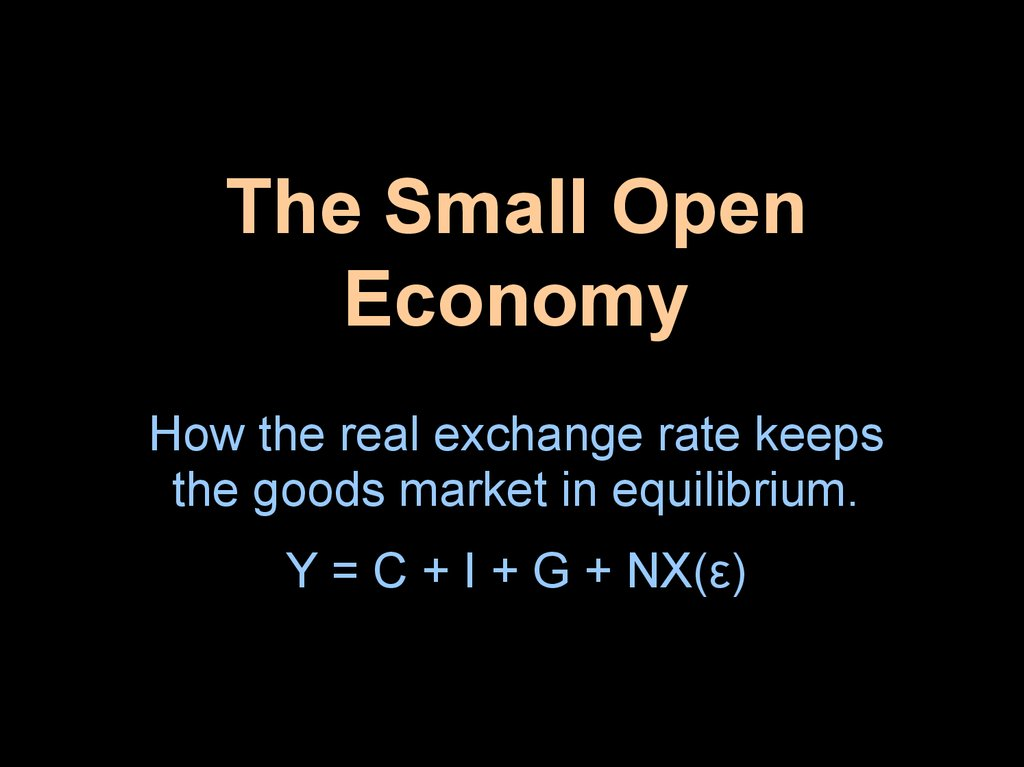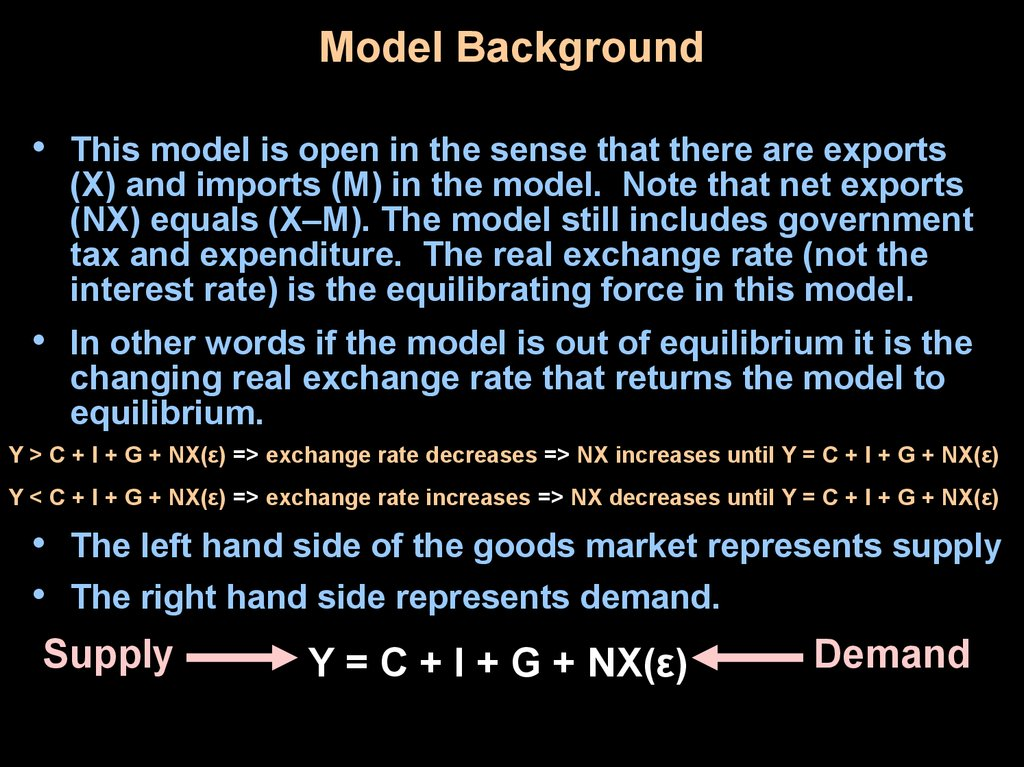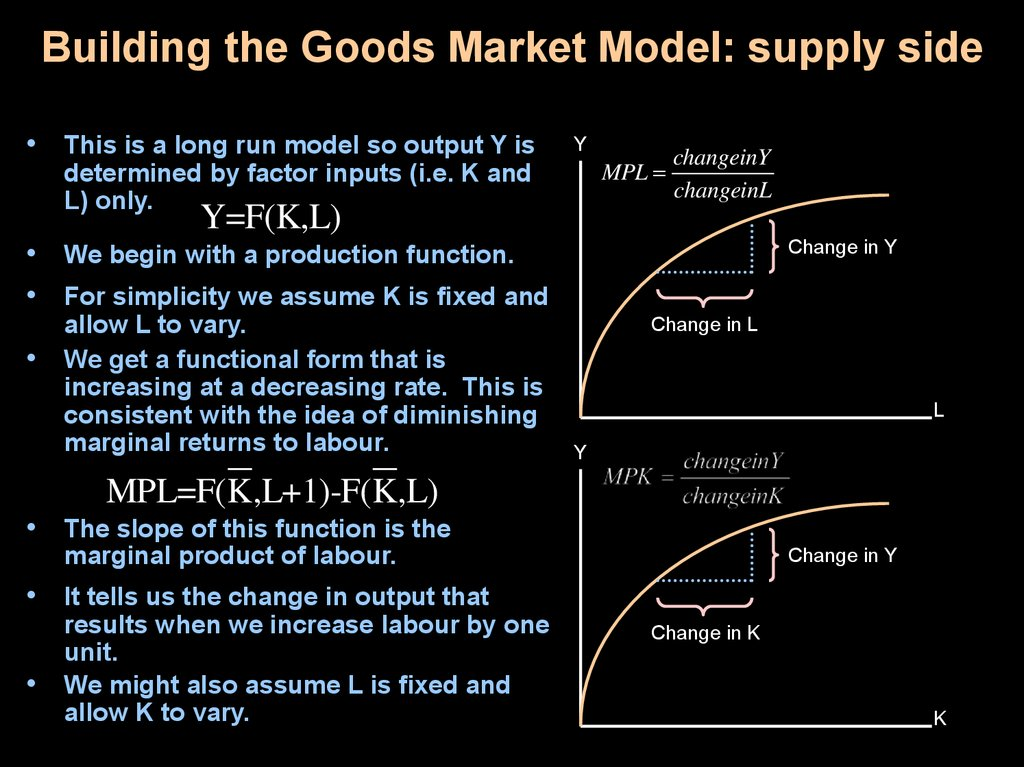# The Small Open Economy

## 1. The Small Open Economy

How the real exchange rate keeps
the goods market in equilibrium.
Y = C + I + G + NX(ε)

## 2. Model Background

• This model is open in the sense that there are exports
(X) and imports (M) in the model. Note that net exports
(NX) equals (X–M). The model still includes government
tax and expenditure. The real exchange rate (not the
interest rate) is the equilibrating force in this model.
• In other words if the model is out of equilibrium it is the
changing real exchange rate that returns the model to
equilibrium.
Y > C + I + G + NX(ε) => exchange rate decreases => NX increases until Y = C + I + G + NX(ε)
Y < C + I + G + NX(ε) => exchange rate increases => NX decreases until Y = C + I + G + NX(ε)
• The left hand side of the goods market represents supply
• The right hand side represents demand.
Supply
Demand
Y = C + I + G + NX(ε)

## 3. Building the Goods Market Model: supply side

• This is a long run model so output Y is
Y
MPL
determined by factor inputs (i.e. K and
L) only.
changeinY
changeinL
Y F ( K , L)
Y=F(K,L)
• We begin with a production function.
• For simplicity we assume K is fixed and
allow L to vary.
We get a functional form that is
increasing at a decreasing rate. This is
consistent with the idea of diminishing
marginal returns to labour.
Change in Y
Change in L
L
Y
Y F ( K , L)
MPL=F(K,L+1)-F(K,L)
• The slope of this function is the
marginal product of labour.
Change in Y
• It tells us the change in output that
results when we increase labour by one
unit.
We might also assume L is fixed and
allow K to vary.
Change in K
K

## 4. Building the Goods Market Model: supply side

If we chose to combine these
images we would get a surface
with output on the vertical axis
and capital and labour on the
other axes.
2
0
10
Labor
6
4
10
8
7.5
Output
5
2.5
0
0
2
4
6
Capital
8
10
Y F ( K , L)
0
In this case a cross section of
the surface would provide us
with the two-dimensional
production functions.
2
4
6
8
10
10
7.5
Output
5
2.5
0
0
2
6
4
Labor
8
10

## 5. Building the Goods Market Model: supply side

• Factor demand is the marginal
Real
Wage
product of that factor. labour
demand, for example, is defined
as the MPL.
• The real wage W/P is the real price
(W/P)*
MPL is
labour
Demand
of labour. Where W (nominal
wage) and P (price) are
determined exogenously.
• To determine the optimal amount
of L, firms add L until the
MPL = W/P.
L*
L
Real
Rental
Rate
• This is the profit maximization
process that ultimately determines
output.
• The process is exactly the same
for capital K. MPK = R/P (rental
rate of capital divided by the price
level).
(R/P)*
MPK is
Capital
Demand
K*
K

## 6. Building the Goods Market Model: demand side

• We begin with consumption, investment, government expenditure, and net exports.
This gives us the following national income accounting identity.
Y = C + I(r*) + G + NX(ε) …We know Y=F(K,L)
• Now, given a savings rate “s” we say c = (1–s) is the marginal propensity to
consume. This gives us a consumption function C = c(Y–T).
• “r” is the real interest rate. Investment and the real interest rate have a negative
relationship so I(r) is negatively sloped. As “r” increases “I” decreases. In this
case however it is the world interest rate (r*) that dominates the small open
economy. Much like a perfect competitor is a price taker the small open economy
is an interest rate taker. Domestic investors always have access to the world
interest rates and their economy is so small it can not affect the world interest rate.
• “T” is the amount of tax collected.
• NX = (X–M) is the trade balance and is dependent on the real exchange rate “ε”
From this we get…
• Y = c(Y–T) + I(r*) + G + NX(ε)
…rearranging we get,
Y – c(Y–T) – G = I(r*) + NX(ε) …or,
Sn = I(r*) + NX(ε)
…or
Sn – I(r*) = NX(ε)
…so national savings – investment = net exports
we call (S–I) net capital outflow because when savings is positive it is lent abroad.

## 7. Goods Market Equilibrium: The Loanable Funds Market

• We said the small open economy
model long run equilibrium occurs
at the point where
Y = c(Y – T) + I(r*) + G + NX(ε) and
that if the system is out of
equilibrium then “ε” must change
to equilibrate the system.
• Recall that S – I(r*) = NX(ε) is just a
rearrangement of the goods
market into net capital flow and
rearrangement is called the
loanable funds market.
ε
S-I(r*)
ε
ε
ε*
NX(ε)
• If the loanable funds market is out
of equilibrium then the exchange
rate adjusts to equilibrate it which
in turn ensures that the goods
market is in equilibrium.
S-I(r*),NX(ε)

## 8. The Markets in Transition

• There are various effects
which can enter the model and
change either S – I or NX
leading to a change in the real
exchange rate.
• Things that might shift S – I
include changes in Y, T, G, or
the mpc.
• Things that might shift NX
include changes in domestic
policies such as tariffs or
quotas.
• All these changes require a
different real exchange rate to
equilibrate the market.
ε
S-I(r*)
S-I(r*)
ε*
NX(ε)
ε*
ε*
NX(ε)
S-I(r*),NX(ε)

## 9. Conclusion

• The small open economy model is a simple
static model that allows us to see how the
real exchange rate adjusts to keep
equilibrium in the loanable funds market
which implies equilibrium in the goods
market. We also see how various
exogenous shocks can affect either (S–I) or
NX and therefore lead to a different real
exchange rate that equilibrates the goods
market.